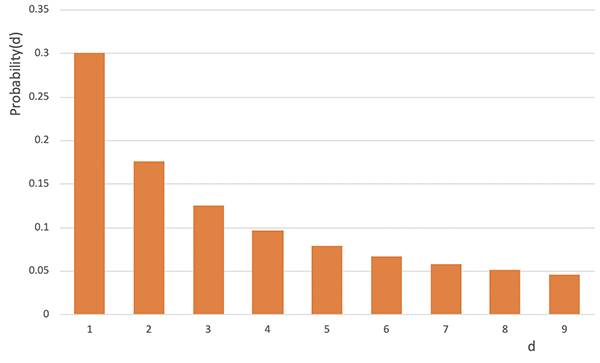# Benfords Law

Written by Paul Bourke
June 2020

For those who have not yet heard of Benfords law, this might interest you as much as it did me. Let's say you looked at 2^n, 3^n, 4*n, 5^n ... for a range of n greater than 0, and looked at the distribution of the first digit (most significant). What do you think it would look like? Or put another way, do you have any reason to believe the first digit would not be equally likely among 1,2,3...9, each digital having 11.1% chance of occurrence?

Note, this can be applied to just one set of powers (31, 32, 33, ...) but the number of samples is quite low since you quickly run into the limits of computer integer representations. To get more samples I suggest forming the statistics including powers of other integers, doesn't actually matter which ones but you might like to preclude pathological cases like 1 and 10.

As it happens the distribution of first digits is anything but uniform. 1 has a probability around 30% and 9 less than 5%. It is known as Benfords law, originally identified circa 1880 when it was noticed that the first few pages of logarithm tables (where leading 1's were) were more worn than the later pages. The equation for the probability of the digit "d" is log10(1+1/d), see graph.I at least found it fascinating, I tested it on a range of things including the Fibonacci series, the integer powers above and populations of the top 1,000 cities. It all makes sense once one realises it arises from distributions with a uniform logarithmic distribution, hence why it applies to lots of natural (fractal) processes. There are some interesting applications to detecting fraud when the fraudster is making up numbers randomly (uniformly) when they should follow Benfords law, and it has been used to convict fraud cases in a court of law.